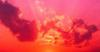### [讨论]换个角度看多态 - Polymorphic Games

2008-10-31 17:31
6597

http://mirror.sweon.net/z0mbie/pgames.txt
可肯能我们写多态时，更多的是考虑等效指令替换，指令乱序，随机指令生成，随机寄存器等这些因素，这篇文章倒是提了另外的思路，所以可以学习一下了，虽然不一定马上加入自己写的东东里，但留个思路日后琢磨吗，不废话了，下面就是.

Polymorphic Games
=================

part 1 -- conditional execution w/o jxx
---------------------------------------

---------

c = condition ? -1 : 0;

; CF <-- condition
sbb ecx, ecx
or
; ecx.high_bit <-- condition
sar ecx, 31

---------

c = a < b ? -1 : 0;

if (a < b) c = -1; else c = 0;

cmp eax, ebx
sbb ecx, ecx

mov ecx, eax
sub ecx, ebx
sar ecx, 31

---------

if (a > b) a = b;

a = a > b ? b : a;

a = a + ((a > b ? -1 : 0) & (b - a))

a += ((b - a) < 0 ? -1 : 0) & (b - a)

sub     ebx, eax
sbb     ecx, ecx
and     ecx, ebx

---------

a = abs(a)
if (a < 0) a = -a;
a = a < 0 ? -a : a;

a = (a ^ (a<0?-1:0)) - (a<0?-1:0)

mov     edx, eax
sar     edx, 31
xor     eax, edx
sub     eax, edx

example 5
---------

if (a != 0)
a = b;
else
a = c;

a = a ? b : c;

a = ((a != 0 ? -1 : 0) & b) | ((a != 0 ? 0 : -1) & c);

cmp     eax, 1
sbb     eax, eax
and     ecx, eax
xor     eax, -1
and     eax, ebx
or      eax, ecx

if (c) a >>= b

a >>= (c ? b : 0),

func_addr = condition ? real_func : fake_func;

int fake_func() { return 0; }

var_addr = condition ? &real_var : &fake_var;

if (condition)
{
statement1;
statement2;
statementN;
}

if (condition) statement1;
if (condition) statement2;
if (condition) statementN;

while(1)
{
if (c) line1;
if (c) line2;
if (c) c = ...;
if (c) line3;
if (c) line4;
}

if (c) if (d) if (e) lineN
- 3个嵌套循环,

if (c==1) line1;
if (c>=2&&c<=3) line2;
if (c==4) line3;

1  难以理解其逻辑
2. 更不容易理解其指令的排列顺序
ok, 可以想象，用相同的C的源代码，来产生不同的一些不同的独特的程序.

#define H0(x)       (((signed)(x)) >> (sizeof((signed)(x))*8-1))
#define H1(a,b)     H0((a)-(b))

#define MIN1(a,b)   ((a)+(H1(b,a) & ((b)-(a))))
#define MIN2(a,b)   ((a)-(H1(b,a) & ((a)-(b))))
#define MIN3(a,b)   ((b)-(H1(a,b) & ((b)-(a))))
#define MIN4(a,b)   ((b)+(H1(a,b) & ((a)-(b))))
//#define MIN5(a,b)   ((a)<(b)?(a):(b))
//#define MIN6(a,b)   ((a)>(b)?(b):(a))
//#define MIN7(a,b)   ((b)>(a)?(a):(b))
//#define MIN8(a,b)   ((b)<(a)?(b):(a))

#define MAX1(a,b)   ((a)+(H1(a,b) & ((b)-(a))))
#define MAX2(a,b)   ((a)-(H1(a,b) & ((a)-(b))))
#define MAX3(a,b)   ((b)-(H1(b,a) & ((b)-(a))))
#define MAX4(a,b)   ((b)+(H1(b,a) & ((a)-(b))))
//#define MAX5(a,b)   ((a)<(b)?(b):(a))
//#define MAX6(a,b)   ((a)>(b)?(a):(b))
//#define MAX7(a,b)   ((b)>(a)?(b):(a))
//#define MAX8(a,b)   ((b)<(a)?(a):(b))

#define ABS1(a)     (((a)^H0(a))-H0(a))
//#define ABS2(a)     ((a)>0?(a):-(a))
//#define ABS3(a)     ((a)>=0?(a):-(a))
//#define ABS4(a)     ((a)<0?-(a):(a))
//#define ABS5(a)     ((a)<=0?-(a):(a))

part 2 -- generating code
-------------------------

if (一些代码已经包含了一些已知的指令)
{
return true
or
仿真这些指令.
}

---------

; at this step we know result of (r1 ? r2)
cmp     r1, r2
jxx     l2
l1:
; never executed really, but can be emulated
{random part of random .code segment}
l2:
; can be either executed or emulated
{part of our code}

---------

; at this step we know that (r1 % (N+1)) == 2
and     r1, N
<jmp|call> dword ptr [offset table1 + r1 * 4]
...
table1:   dd l0
dd l1
dd l2
...
dd lN
l0:
; never executed really, but can be emulated
{random part of random .code segment}
l1:
; never executed really, but can be emulated
{random part of random .code segment}
l2:
; can be either executed or emulated
{part of our code}
lN:
...

1 总体的指令效果是非常接近标志的指令（ps：猜想他的大意应该是说，构造的指令在效果上同真实的、人为写的程序很接近，也就是很

2. 充分和正确的仿真是需要一个真正确定的指令指标（ps:应该就是指对set-of-instructions定义的那些指令）

HWS计划·2020安全精英夏令营来了！我们在华为松山湖欧洲小镇等你

12

0NONAME剑人3 2008-10-31 17:57
2
0
sofa..

===================foxabu13 2008-10-31 19:10
3
0
ps, 用那个PHX Optimized Compiler 跑一下这些东西就没咯 就好比
#define MIN1(a,b)   ((a)+(H1(b,a) & ((b)-(a))))
#define MIN2(a,b)   ((a)-(H1(b,a) & ((a)-(b))))
#define MIN3(a,b)   ((b)-(H1(a,b) & ((b)-(a))))
#define MIN4(a,b)   ((b)+(H1(a,b) & ((a)-(b))))Polymorphic 早期的ASM病毒用的太多了。所以还是把位子占给fg..等待膜拜4
05
0forgot26 2008-11-1 09:31
6
0neineit10 2008-11-1 10:40
7
08
0stalker8 2008-11-2 05:35
9
010
0